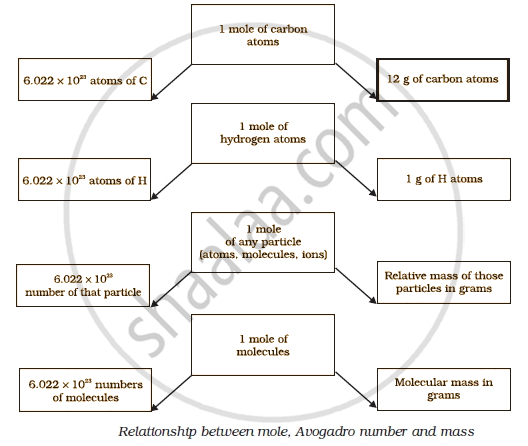Share

# Mole Concept

Course

#### notes

Take an example of the reaction of hydrogen and oxygen to form water:

2H2+ O2 → 2H2O.

The above reaction indicates that
(i) two molecules of hydrogen combine with one molecule of oxygen to form two molecules of water, or
(ii) 4 u of hydrogen molecules combine with 32 u of oxygen molecules to form 36 u of water molecules.

We can infer from the above equation that the quantity of a substance can be characterised by its mass or the number  of molecules. But, a chemical reaction equation indicates directly the number of atoms or molecules taking part in the reaction. Therefore, it is more convenient to refer to the quantity of a substance in terms of the number of its molecules or atoms, rather than their masses. So, a new unit “mole” was introduced. One mole of any species (atoms,molecules, ions or particles) is that quantity in number having a mass equal to its atomic or molecular mass in grams.

The number of particles (atoms, molecules or ions) present in 1 mole of any substance is fixed, with a value of 6.022 ×  1023. This is an experimentally obtained value. This number is called the Avogadro Constant or Avogadro Number (represented by N0),named in honour of the Italian scientist, Amedeo Avogadro. 1 mole (of anything) = 6.022 × 1023 in number,

as, 1 dozen = 12 nos.
1 gross = 144 nos.

Besides being related to a number, a mole has one more advantage over a dozen or a gross. This advantage is that mass of 1 mole of a particular substance is also fixed.

The mass of 1 mole of a substance is equal to its relative atomic or molecular mass in grams. The atomic mass of an lement gives us the mass of one atom of that element in atomic mass units (u). To get the mass of 1 mole of atom of that element, that is, molar mass, we have to take the same numerical value but change the units from ‘u’ to ‘g’. Molar
mass of atoms is also known as gram atomic mass. For example, atomic mass of hydrogen=1u. So, gram atomic mass of hydrogen = 1 g.

#### description

• Relating mole and atomic mass; arriving at gram atomic mass and then gram atom; atomic mass is a number dealing with one atom; gram atomic mass is the mass of one mole of atoms.

• Relating mole and molecular mass arriving at gram molecular mass and gram molecule – molecular mass is a number dealing with a molecule, gram molecular mass is the mass of one mole of molecules.

• Simple calculations based on relation of mole to mass, volume and Avogadro’s number.
If you would like to contribute notes or other learning material, please submit them using the button below.

### Shaalaa.com

Atoms and Molecules (Mole Concepts) [00:22:07]
S
0%